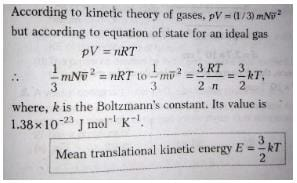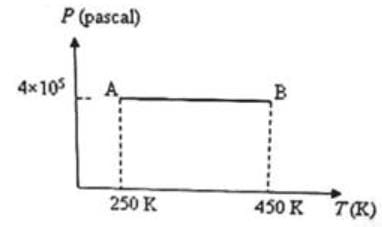NEET  >  Test: Behaviour of Ideal Gases

# Test: Behaviour of Ideal Gases

Test Description

## 10 Questions MCQ Test Physics Class 11 | Test: Behaviour of Ideal Gases

Test: Behaviour of Ideal Gases for NEET 2022 is part of Physics Class 11 preparation. The Test: Behaviour of Ideal Gases questions and answers have been prepared according to the NEET exam syllabus.The Test: Behaviour of Ideal Gases MCQs are made for NEET 2022 Exam. Find important definitions, questions, notes, meanings, examples, exercises, MCQs and online tests for Test: Behaviour of Ideal Gases below.
Solutions of Test: Behaviour of Ideal Gases questions in English are available as part of our Physics Class 11 for NEET & Test: Behaviour of Ideal Gases solutions in Hindi for Physics Class 11 course. Download more important topics, notes, lectures and mock test series for NEET Exam by signing up for free. Attempt Test: Behaviour of Ideal Gases | 10 questions in 10 minutes | Mock test for NEET preparation | Free important questions MCQ to study Physics Class 11 for NEET Exam | Download free PDF with solutions
 1 Crore+ students have signed up on EduRev. Have you?
Test: Behaviour of Ideal Gases - Question 1

### In the case of real gases, the equation of state, PV = RT (where P, V and T are respectively the pressure, volume and absolute temperature), is strictly satisfied only if corrections are applied to the measured pressure P and the measured volume V. The corrections for P and V arise respectively due to

Detailed Solution for Test: Behaviour of Ideal Gases - Question 1

According to kinetic theory of gases there is no intermolecular force of attraction between the molecule of gases but its not true as gases liquifise, at high pressure molecular interaction starts due to which pressure on the walls decreases as molecule dragged back so pressure exerted by real gas is less than ideal gas, due to repulsive forces molecule behave as small spheres and hence volume decreases

Test: Behaviour of Ideal Gases - Question 2

### Which one of the following quantities can be zero on an average for the molecules of an ideal gas in equilibrium?

Detailed Solution for Test: Behaviour of Ideal Gases - Question 2

In case of ideal gases the average velocity is always zero. Hence the average momentum is zero.
Whereas average speed is non- zero so the kinetic energy is also non-zero,  as these two are scalar quantities.

Test: Behaviour of Ideal Gases - Question 3

### Two specific heats of a perfect gas are related by:

Detailed Solution for Test: Behaviour of Ideal Gases - Question 3

When cp & cv are given calorie & R in Joule then Cp – Cv = (R/J)

Test: Behaviour of Ideal Gases - Question 4

One mole of any substance at any temperature, pressure or volume always contains ________ molecules.

Detailed Solution for Test: Behaviour of Ideal Gases - Question 4

1 mole = 6.02 x 1023

Test: Behaviour of Ideal Gases - Question 5

Oxygen and nitrogen in two enclosures have the same mass, volume and pressure. The ratio of the temperature of oxygen to that of nitrogen is:

Detailed Solution for Test: Behaviour of Ideal Gases - Question 5

For same mass the ratio of moles of oxygen to that of nitrogen is 14:16 = 7:8
And we know that PV = nRT
Hence as V and P are also same, ratio of temperature of oxygen to that of nitrogen is inverse of the ratio of moles that is 8:7

Test: Behaviour of Ideal Gases - Question 6

When the temperature goes up, the pressure inside a rigid container will _____.

Detailed Solution for Test: Behaviour of Ideal Gases - Question 6

The pressure law states that for a constant volume of gas in a sealed container the temperature of the gas is directly proportional to its pressure. This can be easily understood by visualising the particles of gas in the container moving with a greater energy when the temperature is increased.

Test: Behaviour of Ideal Gases - Question 7

Four moles of an ideal diatomic gas is heated at constant volume from 20° C to 30° C. The molar specific heat of the gas at constant pressure (Cp) is 30.3 Jmol-1K-1 and the universal gas constant (R) is 8.3 Jmol-1K-1. The increase in internal energy of the gas is

Detailed Solution for Test: Behaviour of Ideal Gases - Question 7

The value of Cp is 30.3
and as Cp-Cv = R(8.3)
hence Cv = 30.3-8.3
Cv is 22
change in internal energy = no of moles × Cv × change in temperature
hence
change in internal energy = 22 × 4 × 10
= 880j
Hence Option D is correct.

Test: Behaviour of Ideal Gases - Question 8

A region of the earth’s atmosphere contains n molecules (treated as ideal gas molecules) per unit volume. The temperature of air in the region is T. If k represents Boltzmann’s constant and R represents universal gas constant, the pressure of air in the region is

Detailed Solution for Test: Behaviour of Ideal Gases - Question 8

PV = nRT
Where n = number of moles = m/NA
So, P = (m/V)(R/NA)T
Also, we know that R/NA = k
So, P = nkT

Test: Behaviour of Ideal Gases - Question 9

The average kinetic energy of translation of a molecule of an ideal gas at temperature T is:

Detailed Solution for Test: Behaviour of Ideal Gases - Question 9Test: Behaviour of Ideal Gases - Question 10

Three moles of an ideal monoatomic gas is initially in the state A shown in the adjoining pressure-temperature graph. It is taken to state B without changing its pressure. If R is the universal gas constant, the work done by the gas in this process isDetailed Solution for Test: Behaviour of Ideal Gases - Question 10

The work done by the gas in taking it from state A to state B = PΔV where ΔW is the increase in volume at constant pressure P.

We have PV = μRT where p is the number of moles in the sample of the gas and R is the universal gas constant.

Therefore we have PΔV = μR ΔT = 3 xR(450 - 250) = 600R

## Physics Class 11

126 videos|449 docs|210 tests
 Use Code STAYHOME200 and get INR 200 additional OFF Use Coupon Code
Information about Test: Behaviour of Ideal Gases Page
In this test you can find the Exam questions for Test: Behaviour of Ideal Gases solved & explained in the simplest way possible. Besides giving Questions and answers for Test: Behaviour of Ideal Gases, EduRev gives you an ample number of Online tests for practice

## Physics Class 11

126 videos|449 docs|210 tests

### How to Prepare for NEET

Read our guide to prepare for NEET which is created by Toppers & the best Teachers